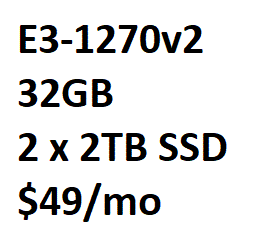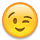#### Howdy, Stranger!

It looks like you're new here. If you want to get involved, click one of these buttons!# PHP question

HI all,

Just a quick one, what's the best way to remove the part before a decimal place and the part after a decimal place in a string.

E.g

string of "12345.567843

I need to return both 12345 and 567843 seperately

• edited August 2013

use the explode function

```\$source  = "12345.567843";
\$result = explode(".", \$source);
echo \$result; // 12345
echo \$result; // 567843
```
• ``````<?php
\$str = "12345.567843";
\$arr = explode(".", \$str);
print_r(\$arr);
``````

Outputs:

``````Array
(
 => 12345
 => 567843
)
``````
• edited August 2013

GOD BLESS OVERKILLING

```preg_match_all('|([0-9]*)\.([0-9]*)|', \$in, \$out);

print_r(\$out);```
Thanked by 3Hassan Spencer fisle
• edited August 2013
``````<?php
preg_match('/([0-9]+)\.([0-9]+)/', \$str, \$regx);
array_shift(\$regx);
print_r(\$regx);
``````
• edited August 2013

But regex's are so slow```\$value = 12345.567843;
\$first_part = floor(\$value);
\$second_part = str_replace("\${first_part}.", '', \$value);
```

In reality I'd probably do:

`list(\$first_part,\$second_part) = explode('.', \$value);`

After first testing that \$value is in the expected format.

• I feel like we should have a competition to see who can do this using the most code.

• It's interesting to see how many different ways a simple task can be accomplished• @super320 said:
<?php
preg_match('/([0-9]+).([0-9]+)/', \$str, \$regx);
array_shift(\$regx);
print_r(\$regx);

I can take a nap while that preg_match is running ;-)

• ``````\$value = "12345.567843";
\$first_part = strtok(\$value, '.');
\$second_part = strtok('.');
``````
• What no sed or awk? This thread would not be complete without it.

• ```\$firstpart = '';
\$lastpart = '';
\$array = array();
\$string = '12345.567843';
\$stringsize = strlen(\$string);

for(\$i=0; \$i<\$stringsize; \$i++)
{
\$array[] = \$string[\$i];
if(\$string[\$i] == '.')
{
\$dotposition = \$i;
}
}

foreach(\$array as \$key => \$val)
{
if(\$key < \$dotposition)
{
\$firstpart .= \$val;
}
elseif(\$key > \$dotposition)
{
\$lastpart .= \$val;
}
}

echo \$firstpart;
echo "\n";
echo \$lastpart;
```
• lol you guys seems bored...• ```\$string = '12345.567843';
\$array = str_split(\$string);
\$dotposition = array_search('.', \$array);
\$firstpart = implode('', array_slice(\$array, 0, \$dotposition-1));
\$lastpart = implode('', array_slice(\$array, \$dotposition+1, count(\$array)-1));

echo \$firstpart;
echo "\n";
echo \$lastpart;
```
• ``````\$value = "12345.567843";
\$value = str_split(\$value);
\$first_part = \$second_part = '';
foreach(\$value as \$key => \$char) {
unset(\$value[\$key]);
if(\$char == '.') {
\$second_part = implode('', \$value);
break;
}
\$first_part .= \$char;

}
``````
• ``````\$value = "12345.567843";
list(\$first_part, \$second_part) = sscanf(\$value, "%d.%d");
``````
• This only removes the decimal. Too lazy to figure out the rest.

`echo '12345.567843' | sed 's/.//g'`

• ```\$firstpart = '';
\$string = '12345.567843';
\$i = 0;

while(true)
{
\$firstpart .= \$string[\$i];
if(\$string[\$i] == '.')
{
break;
}
\$i++;
}

\$lastpart = str_replace(\$firstpart, '', \$string);

echo rtrim(\$firstpart, '.');
echo "\n";
echo \$lastpart;
```
• @sman said:
This only removes the decimal. Too lazy to figure out the rest.

`echo '12345.567843' | sed 's/.//g'`

That removes everything.

I guess that you mean `echo '12345.567843' | sed 's/\.//g'`

Yeah, silly forum engine.• ``````\$value = "12345.567843";
\$arr = array('','');
\$found_dot = 0;
foreach(str_split(\$value) as \$char) {
\$found_dot = \$found_dot || \$char == '.';
\$arr[\$found_dot] .= \$char == '.' ? '' : \$char;
}

list(\$first_part, \$second_part) = \$arr;
``````
• @pskosinski said:
Yeah, silly forum engine.Thanks for catching that. Yea, posted without the {code} tags at first and it must have stripped that.

• `print substr(\$word, 0, strpos(\$word,".")). " ". substr(\$word, strpos(\$word,".")+1);`